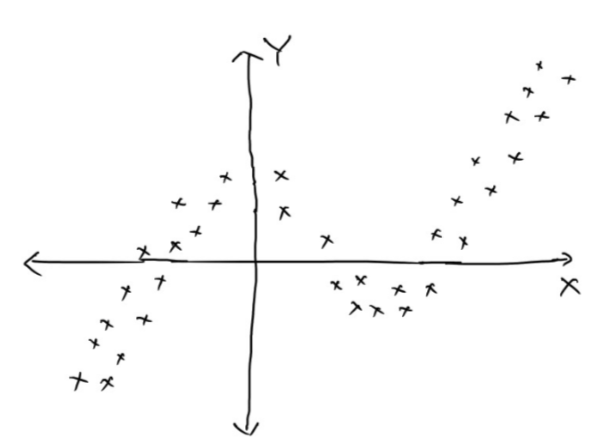Phone

+919997782184

Email

support@roboticswithpython.com

Geeks of Coding

Home Forums Assignment NPTEL_ Introduction to Machine learning Introduction to Machine Learning (IITM) Week 1 Assignment

• Author
Posts
• #540

1)- Which of the following is a supervised learning problem?

Predicting the outcome of a cricket match as a win or loss based on historical data.Recommending a movie to an existing user on a website like IMdB based on the search history (including other users)
Predicting the gender of a person from his/her image. You are given the data of 1 Million images along with the gender
Given the class labels of old news articles, predicting the class of a new news article from its content. Class of a news article can be such as sports, politics, technology, etc

2)- Which of the following are classification problems?
Answer- Predicting if a cricket player is a batsman or bowler given his playing records.
Predicting if a particular route between two points has traffic jam or not based on the travel time of vehicles.

3)- Which of the following is a regression task?</div>
Answer- Predicting the monthly sales of a cloth store in rupees.
Predicting the confirmation probability (in fraction) of your train ticket whose current status is waiting list based on historical data.

4)- Which of the following is an unsupervised task?
Answer- Grouping images of footwear and caps separately for a given set of images

5)- Which of the following is a categorical feature?
Answer – Branch of an engineering student

6)- Let X and Y be a uniformly distributed random variable over the interval [0,4] and [0,3] respectively. If X and Y are independent events, then compute the probability, P(max(x, y)>2)?

7)- Let the trace and determinant of a matrix

|a  ,  b|

|c  ,  d| be 4 and 3 respectively. The eigenvalues of are

8)- What would be the ideal complexity of the curve which can be used for polynomial curve fitting for the data shown below. (y-axis denotes the dependent variable)9)- Which of the following are true about bias and variance of overfitted and under fitted models?

Answer- Underfitted models have high bias

Overfitted models have high variance

10)- What happens when your model complexity increases?